Previous Article in Journal
Energy Harvesting Backpacks for Human Load Carriage: Modelling and Performance Evaluation
Previous Article in Special Issue
Nonlinear Voltage Control for Three-Phase DC-AC Converters in Hybrid Systems: An Application of the PI-PBC Method
Open AccessArticle

# LC Impedance Source Bi-Directional Converter with Reduced Capacitor Voltages

byDogga Raveendhra *,Rached Dhaouadi,Habibur Rehman andShayok MukhopadhyayCollege of Engineering, American University of Sharjah, Sharjah 26666, UAE
*
Author to whom correspondence should be addressed.
Electronics 2020, 9(7), 1062; https://doi.org/10.3390/electronics9071062
Received: 3 June 2020 / Revised: 15 June 2020 / Accepted: 17 June 2020 / Published: 28 June 2020

## Abstract

This paper proposes an LC (Inductor and Capacitor) impedance source bi-directional DC–DC converter by redesigning after rearranging the reduced number of components of a switched boost bi-directional DC–DC converter. This new converter with a conventional modulation scheme offers several unique features, such as a) a lower number of components and b) reduced voltage stress on the capacitor compared to existing topologies. The reduction of capacitor voltage stress has the potential of improving the reliability and enhancing converter lifespan. An analysis of the proposed converter was completed with the help of a mathematical model and state-space averaging models. The converter performance under different test conditions is compared with the conventional bi-directional DC–DC converter, Z-source converter, discontinuous current quasi Z-source converter, continuous current quasi Z-source converter, improved Z-source converter, switched boost converter, current-fed switched boost converter, and quasi switched boost converter in the Matlab Simulink environment. MATLAB/Simulink results demonstrate that the proposed converter has lesser components count and reduced capacitors’ voltage stresses when compared to the topologies mentioned above. A 24 V to 18 V LC-impedance source bi-directional converter and a conventional bidirectional converter are built to investigate the feasibility and benefits of the proposed topology. Experimental results reveal that capacitor voltage stresses, in the case of proposed topology are reduced by 75.00% and 35.80% in both boost and buck modes, respectively, compared to the conventional converter circuit.

## 1. Introduction

The study, development, and applications of bidirectional power converters are gaining a lot of attention due to their vital role in areas like renewable energy systems, DC microgrids, hybrid energy storage systems, smart mobility, etc. A bidirectional DC-DC converter (BDC) allows power flow in both directions. This functionality is not available in a traditional unidirectional DC-DC converter. Because of this flexibility, BDCs are widely used in several applications, such as battery-powered electric vehicles (BEVs) or hybrid electric vehicles (HEVs), power trains, uninterruptable power supplies (UPS), smart grids, charging stations for BEVs and plug-in hybrid electric vehicles (PHEV), aerospace, defense, aerospace, and non-conventional energy sources such as photovoltaic (PV) arrays, fuel cells (FCs), and wind turbines. Specifically, BDCs are widely adopted by the electric vehicle industry to achieve objectives, such as battery charging/discharging and energy recovery during regeneration modes of operation in electric vehicles. In case of the BEVs, electric energy needs to flow in both directions, i.e., from the motor to the battery and vice versa in regenerative mode. To avoid pollutant emissions, the electric vehicle must be powered only by batteries or other electrical sources (fuel cells, solar panels, etc.) [1,2,3,4]. In all the above-mentioned applications, a BDC is preferred for saving space by eliminating a separate boost and buck converter. A BDC can offer some benefits, like cost reduction, improved power density, and effective utilization of the converter . Figure 1 shows the typical structure of the bidirectional DC–DC converters. The BDC, shown in Figure 2, helps to enhance the system efficiency and performance by interfacing with power and energy storage devices . It also avoids a couple of individual unidirectional converters for achieving bidirectional power flow. The BDC’s mode of operation (buck or boost) is mainly decided by power flow direction and voltage levels of sources/energy storage elements. Accordingly, the controller must be designed to regulate the voltage/current of the system. While designing DC–DC converters, the main functional objectives are high power density and high efficiency. The high density can be achieved by increasing the switching frequency  due to the reduction in reactive components size. However, the problem is that increasing the switching frequency increases the switching losses, which leads to efficiency reduction. This problem can be addressed by adopting wide-bandgap power devices along with suitable gate drivers instead of conventional Si devices.
In general, conventional step-up DC–DC converters are classified into isolated and non-isolated converters. Isolated converters like fly-back, push–pull, forward, half-bridge, and full-bridge converters have a high voltage gain by keeping a high enough transformer turns ratio. However, there is a problem with voltage spikes due to transformer leakage inductance, which leads to high power losses across the switch. On the other hand, in non-isolated converters, a high duty cycle is required to get a high voltage gain, which leads to decreasing efficiency due to reverse recovery problems . In addition, non-isolated converters also have the problem of voltage stress nearly equal to the output voltage, causing a reduction of the device’s reliability. Many DC–DC converter topologies are introduced to mitigate the problems mentioned above, such as interleaving topologies for the reduction of current ripple [8,9], soft-switching techniques to mitigate voltage spikes and efficiency improvement , and cascading boost converters  and incorporating a coupled inductor  in the conventional boost topology to get a high conversion gain. Input current ripples are reduced with the help of an interleaving concept, which leads to improving the source life. Additionally, it offers the flexibility of current sharing to enhance the power handling capacity [8,9].
On the other hand, several other converter topologies are suggested in the literature; most of these are designed to meet the various objectives, such as reliability, capacitor voltage reduction, and input current ripple reductions, by placing an impedance network between input DC source and switching network in various fashions. An X-shaped LC impedance network, as shown in Figure 3a, is placed to get the voltage boosting capability by operating a switching network in the shoot-through mode . As an alternative to the Z-source converter, the same authors proposed a quasi Z-source (qZS) converter in two variants based on input current, namely continuous input current q-ZS (qZS-CC) and discontinuous input current q-ZS (qZS-DC) [14,15] with a reduced current and capacitor voltage stresses, respectively. The main variation between these two topologies is the input side inductor connection with the supply. In case of qZS-CC, the inductor is placed directly in series with the source, and it tries to always maintain constant input current, whereas the source current is of discontinuous nature in the case of qZS-DC, which increases the stress on the source . Later, Yu Tang et al. proposed an improved Z-source (IZS) converter  with reduced capacitor stresses. In this paper, the authors claim that the utilization of a low voltage capacitor reduces the inrush current, the resonance between the Z-source inductor and capacitors, and the cost and volume of the system compared to a conventional Z-source converter . The switched boost converter is proposed with a reduced passive components count, achieved by replacing one pair of LCs with power semi-conductor devices to have the same kind of buck-boost conversion, as shown in Figure 3b . However, this topology uses more power semiconductor devices compared to the topologies mentioned above.
A SL-ZS converter is proposed with an enhanced gain by placing switched inductors instead of inductors in the impedance network . However, this topology suffers from a large component count (six power diodes and two inductors higher than the ZS converter) in the switching network. Alternatively, the SL-qZS converter proposed in , consists of switched inductors in place of standard inductors in the qZS converter to reduce the capacitor voltage and startup inrush current compared to the SL-ZS converter. However, the downside of this topology is a higher component count. Hossein Fathi et al.  proposed an enhanced boost ZS converter (EB-ZSC), achieved by replacing the impedance network with switched impedance to enhance the conversion gain further. Although this topology increases gain, it suffers from a higher component count (four inductors, four capacitors, and five power diodes). Additionally, this topology suffers from the usage of sophisticated control platforms to achieve smoother voltage control in the case of adjustable speed-controlled drive applications. Moreover, with a similar concept of variations in the impedance network either in ZS or qZS as discussed above, there are several other impedance source topologies, such as a diode-assisted qZS (DA-qZS) converter , a capacitor-assisted qZS (CS-qZS) converter , and an enhanced boost quasi ZS (EB-qZS) converter , which are proposed in the literature. Though these topologies are mainly proposed for DC–AC power conversion applications due to high reliability (operation during shoot-through mode), they are equally applicable for bi-directional applications and are widely used in micro/nano-grid applications [18,23].
For most of these topologies, it has been suggested to incorporate switched-inductor, switched-capacitor, and hybrid switched-capacitor/switched-inductor structures resulting in high boosting factors. However, the effect of nonlinearity can be increased by increasing the energy storage elements in the circuit, which leads to a higher output current and voltage distortion . Additionally, introducing more energy storage elements in the circuit affects the control complexity, total cost, size, volume, losses, and weight of the converter [25,26]. Moreover, these topologies are suffering from the usage of more capacitors and higher capacitor voltage stresses. Additionally, the voltage across most of the capacitors is generally more than the supply voltage in the case of impedance source topologies in order to perform the voltage boost functionality. Hence, high-voltage Z-capacitors should be used, which may increase the volume and system cost. Capacitors are prone to failure in the field operation of power electronic converters . Hence, due to the stricter reliability constraints brought by aerospace, automotive, defense, space, and energy industries, the stresses and usage of capacitors should be reduced to enhance the converter’s reliability . Therefore, to enhance the life and converter reliability, either reduction in capacitors usage or voltage stresses on the capacitor is highly recommended [29,30].
In this paper, the LC impedance bi-directional DC–DC converter (LC-BDC) is proposed by placing one inductor between source and half-bridge, and one capacitor between source and the load, as shown in Figure 4 . These small passive components are arranged in such a way that the converter offers several features, such as lower capacitor voltages, which in turn reduces the cost, size, and volume of the converter and also increases the reliability while achieving the desired functionality. This topology reduces the voltage stresses on the device due to the usage of small passive components compared to existing converters in the case of SiC converters, which are less immune to parasitic components. The paper is organized as follows: the working principle, modes of operation, mathematical modeling, and state-space average models of the proposed topology are discussed in Section 2. The concept validation using simulation and experimentation, along with the respective results, are presented in Section 3. Additionally, to demonstrate the effectiveness of the proposed topology, a detailed comparative analysis of the proposed converter and conventional converter is carried out along with the results of the proposed converter. Moreover, a separate simulation-based comparative analysis of the proposed LC converter with eight similar boost/buck-boost converter topologies is presented in Section 4. Finally, conclusions are presented in Section 5 of this paper.

## 2. Proposed System

The LC bidirectional converter shown in Figure 4 is an advanced version of a conventional bidirectional converter and switched boost bidirectional converter, designed to reduce the voltage stresses on the capacitor. The primary function of the inductor is to store energy during the converter “on” period and release the stored energy during the “off” period of the primary device. The inductor is also used to eliminate the current ripple. Another energy storage element, the capacitor, is used to eliminate the ripple in the output voltage in both cases, namely the conventional BDC and the proposed BDC. Switches M1 and M2 are unidirectional switches used to realize the bidirectional power flow in the test setup which operate in a complementary fashion. For the forward direction of power flow, M1 must be in the “on” state, and S1 acts as the main switch operating at switching frequency, while D2 acts as a freewheeling diode. Similarly, M2 must be in the “on” position for the reverse direction of power flow, and S2 acts as the main switch, which operates at switching frequency, while D1 acts as a freewheeling diode. VLV and RHV are source and load in boost mode, and VHV and RLV are source and load in buck mode. The gating signals for boost switch (G1) and buck switch (G2) complement each other. The duty cycle of boost switch (S1) and buck switch (S2) is denoted as $δ 1$ and $δ 2$, respectively. Ts represents the switching period of switches S1 and S2.

#### 2.1. Boost Operation

The equivalent circuit and idealized waveforms in boost mode of the LC-BDC converter are depicted in Figure 5 and Figure 6, respectively. The converter operation is considered to be in boost mode, during which the switch (S1) is pulse-modulated and the diode D2 freewheels. The boost mode operation is further categorized into two sub-modes of operation over a switching period, and the equivalent circuit of each sub-mode is depicted as shown in Figure 7.

#### 2.1.1. Mode 1 (t0 < t < t1): (S1 ON, D2 OFF)

In this mode, switch S1 is turned on by applying a gate signal. The inductor L starts charging linearly through switch S1, and the capacitor C will discharge through load RHV. Hence, the diode D2 goes into the “off” state. The equivalent circuit during this mode of operation is shown in Figure 7a. The current through the inductor L($i L$) and the voltage across the capacitor C are given by
$V L = V L V = L d i L d t$
$i L ( t ) = V L V L ( t − t 0 ) + i L ( t 0 )$
$i C = − v H V R H V$
$v C ( t ) = i H V ( t ) R H V − V L V$
This mode of operation ends when the gate pulses to switch S1 are withdrawn.

#### 2.1.2. Mode 2 (t1 < t < t2): (S1 OFF, D2 ON)

At the instant when the gate pulses of switch S1 are removed, the switch S1 goes into the “off” state due to which the voltage across the inductor brings the diode D2 into the forward-biased state. The equivalent circuit during this mode is shown in Figure 7b. In this mode, both inductor and source feed power to the load, and the inductor charges the capacitor. Hence, there is a formation of the LC tank in this mode, which can offer zero voltage switching to the upper switch with the proper selection of the snubber capacitor. In this mode, the current through L($i L$) reaches its minimum value. The current flowing through the inductor L($i L$) and the voltage across the capacitor C are given by
$V L = V L V − V H V = L d i L d t$
$i L ( t ) = V L V − V H V L ( t − t 1 ) + i L ( t 1 )$
$v C ( t ) = i H V ( t ) R H V − V L V$
$i C c ( t ) = i L ( t ) − i H V ( t )$
This mode ends at t = Ts when the gate signal is provided to S1 in the next switching cycle. Similar operation (Mode 1 and Mode 2) continues for several switching cycles until a power flow is required in the forward direction

#### 2.2. Buck Mode of Operation of LC-BDC Converter

BDC operates in buck mode when there is a requirement of power flow in the reverse direction, and its equivalent circuit is shown in Figure 8. The converter operation is considered to be in reverse buck mode, during which the switch S2 is pulse-modulated and the diode D1 in a freewheeling mode. The buck mode of operation is further categorized into two sub-modes (i.e., mode 3 and mode 4) of operation over a switching period. The operating mode from mode 3 to mode 4 in buck mode is similar to the mode 2 to mode 1 of the boost mode of operation, respectively. Figure 9 illustrates the characteristic waveforms of the converter in buck mode, and its equivalent circuits in each sub-mode are depicted as shown in Figure 10.

#### 2.2.1. Mode 3 (t3 < t < t4): (S2 ON, D1 OFF)

This mode starts at t = t3 when the gate signal is given to S2. At this instant, the main switch S2 comes into conduction, and the diode D1 goes into the “off” state. The supply VHV then directly energizes the inductor L. The capacitor is also discharged through the inductor. It leads to the formation of the LC tank, as shown in Figure 10a, similar to mode 2. This feature offers the resonating switching functionality to the upper switch. The current flowing through L($i L$) and the voltage across capacitor C ($v C$) are given as
$v L b ( t ) = V H V − v L V$
$i L b ( t ) = V H V − v L V L ( t − t 3 ) + i L b ( t 3 )$
$v C ( t ) = V H V − i L V ( t ) R L V$
$i C ( t ) = i i n b ( t ) − I L b ( t )$
This mode continues until the gate pulse of S2 is withdrawn at t = t4.

#### 2.2.2. Mode 4 (t4 < t < t5): (S2 OFF, D1 ON)

This mode starts at t = t4 when the gate pulses to the main switch are removed. Hence S2 goes into the “off” state. The voltage across the inductor brings diode D1 into “on” state, and it continues until t = t5. The energy stored in inductor L discharges through the load. The capacitor charges from the source. During this mode, the current flowing through the inductor L and voltage across the capacitor C can be expressed as
$v L b ( t ) = − i L V ( t ) R H V$
$i L b ( t ) = − v L V L ( t − t 4 ) + i L b ( t 4 )$
$v C ( t ) = V H V − i L V ( t ) R L V$
$i C ( t ) = i i n b ( t )$
This mode ends at t = Ts when the gate signal is given to S2 in the next switching cycle. Similar operation of mode 3 and mode 4 continues, for several switching cycles, until power flow is required in the reverse direction.

#### 2.3. State Space Analysis

This section presents the development of a small-signal AC model followed by the derivation of the state-space model equations for one complete switching cycle. For this analysis, few assumptions are considered; (i) the converter is operating in continuous conduction mode, and (ii) there is no trace resistance. For the proposed converter, the state variables are the current through the inductor iL and the voltage across the coupling capacitor VC. A complete derivation of the state-space model and small-signal analysis for boost mode is presented. A similar derivation method can also be used for buck mode. With the inclusion of the parasitic components during both “on” and “off” states, the system can be represented with the help of the state-space model as follows.
$[ d i L d t d v C d t ] = [ − ( r o n + r L ) L 0 0 − 1 C ( R + r C ) ] [ i L v C ] + [ 1 L 0 0 − R ( R + r C ) C ] [ V L V i L o a d ]$
during “off” state:
$[ d i L d t d v C d t ] = [ ( − R r c ( R + r C ) − r L ) 1 L − R ( R + r C ) 1 L R ( R + r C ) C 1 ( R + r C ) C ] [ i L v C ] + [ 1 L R r c ( R + r C ) 0 − R ( R + r C ) ] [ V L V i L o a d ]$
Here, $r o n$—on-state resistance of switching device, $r L$—the equivalent series resistance of the inductor, and $r C$—equivalent series resistance of the capacitor.
The state-space average model of the converter can be written as follows.
$x . = [ A 1 δ 1 + A 2 ( 1 − δ 1 ) ] x + [ B 1 δ 1 + B 2 ( 1 − δ 1 ) ] U$
Here, $x = [ i L v c c ]$, $A 1 = [ − ( r o n + r L ) L 0 0 − 1 C ( R + r C ) ]$, $B 1 = [ 1 L 0 0 − R ( R + r C ) C ]$, $A 2 = [ ( − R r c ( R + r C ) − r L ) 1 L − R ( R + r C ) 1 L R ( R + r C ) C 1 ( R + r C ) C ]$, $B 2 = [ 1 L R r c ( R + r C ) 0 − R ( R + r C ) ]$, $u = [ V L V i L o a d ]$
Define:
$δ 1 T s = t 1 − t 0 & t 2 − t 1 = ( 1 − δ 1 ) T s$
The duty ratio of the main switch S1 is defined as
$δ 1 = t 1 − t 0 t 2$
The turn-off duty cycle of the main switch S1 is
$δ ′ 1 = t 2 − t 1 t 2$
Substituting the duty ratio values from (20)–(22) in Equations (17)–(19) and then incorporating the perturbation effect into the state variables and other variables around the steady-state values gives
$i L = I L + i ^ L , v L V = V L V + v ^ L V , v C = V C + v ^ C , v H V = V H V + v ^ H V , δ 1 = D 1 + d 1$
where D1 is the duty ratio of the main switch under steady-state condition. After solving the above state-space equation, the steady-state gains of the converter can be obtained as
$V C = D 1 V L V 1 − D 1 & I L = I H V 1 − D 1$
Comparing small-signal AC parameters while ignoring the considerably very small second-order quantities, and then solving the equations gives the following two transfer functions.

#### 2.3.1. Control-to-Output Transfer Function

From the small-signal AC model, the control-to-output (output voltage to duty ratio) transfer function can be found under the condition of $v ^ i n = 0 & i ^ L = 0$, which is shown in Equation (25).
$v ^ o 1 − d ^ 1 = [ − R ( R + r c ) × ( 1 + C S r c ) × ( R V L V r L − R 2 V L V D 1 2 − R 2 V L V + R V L V r o n + V L V r L r c + V L V r c r o n + 2 R 2 V L V D 1 + 2 I l o a d R 2 r L + I l o a d R 2 r c + I l o a d R 2 r o n − 2 I l o a d R 2 D 1 ( r L + r c ) + I l o a d R 2 D 1 2 r c − I R l o a d 2 D 1 2 r o n + L R S V L V + L S V L V r c + 2 I l o a d R r L r c + I l o a d R r c r o n + I l o a d L R 2 S + I l o a d L R S r c − 2 I l o a d R D 1 r L r c − I l o a d L R 2 S D 1 − I l o a d R D 1 2 r c r o n − I l o a d L R S D 1 r c ) ] [ ( R r L + R r c + r L r c − 2 R 2 D 1 + R 2 + R 2 D 1 2 − R D 1 r c + R D 1 r o n + D 1 r c r o n ) × ( C L R 2 S 2 − C R 2 S D 1 r c + C r o n R 2 S D 1 + C R 2 S r c + C r L R 2 S + R 2 D 1 2 − 2 R 2 D 1 + R 2 + 2 C L R S 2 r c − C R S D 1 r c 2 + 2 C r o n R S D 1 r c + C R S r c 2 + 2 C r L R S r c + L R S − R D 1 r c + r o n R D 1 + R r c + r L R + C L S 2 r c 2 + C r o n S D 1 r c 2 + C r L S r c 2 + L S r c + r o n D 1 r c + r L r c ) ]$
By neglecting parasitic components, it can be simplified as
$v ^ H V 1 − d ^ 1 = R V L V − L S V L V − 2 R V L V D 1 + R V L V D 1 2 − I l o a d L R S + I l o a d L R S D 1 ( D 1 − 1 ) 2 ( C L R S 2 + L S + R D 1 2 − 2 R D 1 + R ) ⇒ v H V 1 − d ^ 1 = R V L V ( 1 − D 1 ) 2 − L S V L V − ( 1 − D 1 ) I l o a d L R S ( D 1 − 1 ) 2 ( C L R S 2 + L S + R ( 1 − D 1 ) 2 )$

#### 2.3.2. Control-to-Input Transfer Function

From the small-signal AC model, the inductor current-to-control (input current to duty ratio) transfer function can be found under the condition of $v ^ L V = 0 & i ^ L o a d = 0$, which is shown in Equation (27).
$i ^ i n 1 − d ^ 1 = − ( R + r c ) ( 2 R 2 V L V − I l o a d R 3 D 1 2 − R V L V r c − I l o a d R 3 + V L V r c r o n + 2 I l o a d R 3 D 1 + 2 R 2 V L V D 1 + I l a o d R 2 r L + I l o a d R 2 r o n + I l a o d R r L r c + I l o a d R r c r o n − C R 3 S V L V D 1 − C I l o a d R 3 S r L − C I l a o d R 3 S r C − C R 2 S V L V r L − C R S V L V r c 2 − 2 C R 2 S V L V r c + C S V L V r c 2 r o n − C I l a o d L R 3 S 2 − C L R 2 S 2 V L V + C R 3 S V L V V D 1 2 − C I l o a d L R 2 S r C − C I l o a d R 3 S D 1 2 r C + C I l o a d R 3 S D 1 2 r o n − C R S V L V r L r C + C R S V L V r c r o n − C L R S 2 V L V r c + 2 C I l o a d R 3 S D 1 r L + 2 C I l o a d R 3 S D 1 r C + C R 2 S V L V D 1 r c + C I l o a d R S r L r c 2 + C I l o a d R S r c 2 r o n + C I l o a d R 2 S r c r o n + C I l o a d L R 3 S 2 D 1 + 2 C I l o a d R 2 S D 1 r L r c + C I l o a d L R 2 S 2 D 1 r c + C I l o a d R 2 S D 1 2 r o n r + R V L V r o n ) [ ( R r L + R r c + r L r c − 2 R 2 D 1 + R 2 + R 2 D 1 2 − R D 1 r c + R D 1 r o n + D 1 r c r o n ) × ( C L R 2 S 2 − C R 2 S D 1 r c + C r o n R 2 S D 1 + C R 2 S r c + C r L R 2 S + R 2 D 1 2 − 2 R 2 D 1 + R 2 + 2 C L R S 2 r c − C R S D 1 r c 2 + 2 C r o n R S D 1 r c + C R S r c 2 + 2 C r L R S r c + L R S − R D 1 r c + r o n R D 1 + R r c + r L R + C L S 2 r c 2 + C r o n S D 1 r c 2 + C r L S r c 2 + L S r c + r o n D 1 r c + r L r c ) ]$
By neglecting parasitic components, it can be simplified as
$i ^ i n 1 − d ^ 1 = 2 V L V − I l o a d R ( 1 − D 1 ) + C R V L V S ( 1 − D 1 ) ( C L R S 2 + L S + R D 1 2 − 2 R D 1 + R ) ⇒ i i n 1 − d ^ 1 = R V L V ( 1 − D 1 ) 2 − L S V L V − I l o a d ( 1 − D 1 ) ( 1 − D 1 ) ( C L R S 2 + L S + R ( 1 − D 1 ) 2 )$

#### 2.3.3. Step and Bode Responses of LC-BDC

From the derived transfer functions, the step responses of various variables are presented in Figure 11. From these results, it can be understood that the variations in input currents and capacitor voltages for both line and load disturbance are low in the case of the proposed converter compared to the existing converter. Moreover, the step responses reveal that the proposed LC-BDC converter response is the same for inductor current and capacitor current and capacitor voltage against the duty ratio, whereas input current transients against duty ratio variations are reduced in LC-BDC. It can also be noted that in the case of supply variations, the transient responses of the inductor current, output voltage, load current, and capacitor voltage are improved.

#### 2.3.4. Ripple Capacitor Voltage

From the charge balance equation and further simplification of the above Equation (25) in the steady-state, the capacitor ripple voltage can be calculated as
$Δ v C c b o o s t = D 1 V H V C R F S$
From (29), the capacitor value can be sized to minimize the voltage ripple across the capacitor.

## 3. Experimental Results and Discussion

The proposed LC impedance bi-directional dc-dc converter has been successfully validated through experiments in both boost and buck modes. The parameters considered for the experimental validations are summarized in Table 1. The experimental setup of the proposed converter is shown in Figure 12. The system performance is evaluated in both steady-state and transient conditions while feeding power to two series-connected 12 V, 50 W lamp load under various test conditions for the 18 V DC to 24 V DC conversion in forwarding boost mode, and 24 V DC to 18 V DC conversion in reverse buck mode. The inductor current, load current, and capacitor voltage waveforms are captured in both boost and buck modes for both conventional and proposed converters. Comparative analysis through experimental results was carried out, as explained below. In the case of the conventional converter, there is a need for two capacitors (CHV plays a vital role in boost mode, and CLV plays a vital role in buck mode), whereas, in the case of the proposed converter, there is a need for only one capacitor C, which can take care of the functionality of the above mentioned two capacitors in the respective modes. It can be observed that two capacitors are used in the realization of the conventional converter, whereas only one capacitor is used for the realization of the proposed LC-BDC, as shown in Table 1.
The gate signal of the lower switch, inductor current, load current, and capacitor voltage for four switching cycles are captured and presented in Figure 13, Figure 14, Figure 15 and Figure 16. From Figure 14, it can be seen that the peak value of the inductor current is 6.17 A in the conventional converter, whereas it is 6.07 A in the proposed converter for the same load current, as shown in Figure 15. From Figure 16, it can be seen that the voltage across the capacitor is 23.20 V in the case of the conventional converter, whereas it is 5.10 V in the case of the proposed converter. Hence, there is 78.02% of capacitor voltage reduction in the proposed converter as compared to the conventional converter for the same input/output voltage conversion.
In Figure 17, Figure 18 and Figure 19, respectively, a zoomed view of respective parameters is presented during both “on” and “off” state. From these figures, peak values during both transient and steady-state can be measured as listed in Table 2.
Form Table 3, it can be observed that the proposed converter not only offer its best performance during steady-state conditions but also the exhibits same best performance during transient conditions in terms of capacitor voltage stresses.
For the ripple content investigation, a zoomed view of the inductor current, load current, and capacitor voltages are presented in Figure 20, Figure 21, Figure 22 and Figure 23, respectively. The summary of the ripple content for both converter topologies is tabulated in Table 3. From this table, it can be understood that there is a 0.4% reduction of ripple content in capacitor voltages and inductor current for the same content of load current ripples.
For the reverse buck mode of operation, the gating signal of the upper switch, inductor current, load current, and capacitor voltage for four switching cycles have been captured, as presented in Figure 24, Figure 25, Figure 26 and Figure 27. From Figure 25, it can be seen that the peak value of the inductor current is 3.61 A in the case of conventional converter, whereas it is 3.62 A in the case of the proposed converter for the same load current as shown in Figure 26. From Figure 27, it can be seen that the voltage across the capacitor is 16.40 V in the case of conventional converter, whereas it is 7.80 V in the case of the proposed converter. A capacitor voltage reduction of 35.80% can be witnessed in this mode of operation.
For the critical investigation, results have been captured under various test conditions to assess the proposed converter suitability for various applications like smooth turn-on, faster load turn-off, and converter on- and off-switching with variable duty. During these conditions, captured inductor current, load current, and capacitor voltage are shown in Figure 28, Figure 29 and Figure 30, respectively. Moreover, in these figures, a zoomed view of respective parameters is presented during both turn-on and turn-off. From these figures, peak values during both transient and steady states can be measured as listed in Table 4. Form the Table 4, and it can be observed that the proposed converter not only offers its best performance during steady-state conditions but also exhibits the same best performance during transient conditions in terms of capacitor voltage stresses.
For the ripple content investigation, a zoomed view of the inductor current, load current, and capacitor voltages are presented in Figure 31, Figure 32, Figure 33 and Figure 34, respectively. The summary of the ripple content in both the converters is tabulated in Table 5. From this table, it can be extracted that there is a 0.3% reduction of ripple content in capacitor voltages ripples for the same load current.

## 4. Comparative Analysis

Another set of simulations is performed to investigate the performance of the above topologies, and simulation parameters are listed in Table 6 as mentioned below. By using these sets of simulations, various performance parameters such as voltage gains, capacitor voltages, and losses were investigated. These simulations are carried out for various output voltages ranging from 36 V to 108 V with the dc input voltage of 24 V, i.e., voltage gain ranging from 1.5 to 4.5. With the help of this data, a comparative analysis is presented in the following subsections.

#### 4.1. No. of Components

As mentioned earlier, by changing the impedance network configurations, various topologies are proposed, and hence each topology has a different number of components. The number of components used for different topologies are listed and presented as a bar chart shown in Figure 35. From this chart, it is clear that the proposed converter and conventional BDC require fewer components compared to other topologies.

#### 4.2. Capacitor Voltage Stress

Since capacitors used in various topologies are different, total voltage stresses in all capacitors are calculated for comparison purposes. Here in Figure 36, the total capacitor stresses are plotted, while 24 V DC is converted into a range of DC voltage ranging from 36 V to 108 V. From this figure, it is clear that capacitor stresses are low in the case of the proposed converter.

#### 4.3. Efficiency Analysis and Loss Comparison

To perform the converter efficiency analysis, the parasitic resistance of inductors and capacitors, and the diode forward conduction losses are considered in this paper. The parasitic resistance of inductor and capacitor are $r L$ and $r C$, respectively, and forward conduction loss of diode due to forward voltage ($V F$) was assumed to be the same in all topologies for comparative analysis. The impact of the parasitic resistances and the forward voltage drop of the main power devices (MOSFETs) are also considered in this manuscript. Equivalent circuits of all the considered buck-boost bi-directional converters with the inclusion of various parasitic components are presented in Figure 37 for the efficiency calculations. Formulas derived for losses and efficiencies are presented in Table 7.
By using the above-derived formulas, the efficiencies and non-ideal voltage conversion ratios of each topology with respect to gain are presented in Figure 37 and Figure 38, respectively. From these results, it can be observed that the efficiency is higher in conventional BDC and LC-BDC compared to other existing topologies. Moreover, it can be seen that the voltage conversion ratio is more linear in the case of conventional BDC, and the proposed converter compared to other existing topologies. In all existing topologies (except conventional BDC), it can be noted that the performance of the converter is becoming poor as the gain is increased further from the designed gain value.

## 5. Conclusions

In this paper, a new LC bi-directional DC–DC converter utilizing small passive components has been proposed and successfully validated. Experimental results proved that there is a reduction of 75% and 35.8% in capacitor voltage for 24 V to 18 V conversion in boost mode, and 18 V to 24 V conversion in buck mode, respectively. Moreover, there is a reduction of one capacitor compared to conventional BDC for the same conversion. In this paper, the proposed converter performance in both transient and steady-state conditions is investigated and presented. This investigation reveals that the proposed converter is able to offer superior performance in both transient and steady-state conditions. Moreover, a comparative analysis of the proposed converter with the conventional BDC, Z-source converter, discontinues current quasi Z-source converter, continues current quasi Z-source converter, improved Z-source converter, switched boost converter, current-fed switched boost converter, and quasi switched boost converter is presented. This comparative analysis proved that the proposed converter offers superior performance compared to existing converters for the same conversion ratio.

## Author Contributions

Idea, Conceptualization, Formal Analysis, Investigation, Methodology, Software, Validation, and Writing—Original Draft are the main contributions of D.R.; Funding acquisition, Project administration, Resources, Validation, Visualization and Writing—review & editing are the main contributions of R.D.; H.R. and S.M. contributed in terms of Validation and Writing—review & editing. All authors have read and agreed to the published version of the manuscript.

## Funding

This work was supported by the Petrofac Research Chair in Renewable Energy Endowment Fund at the American University of Sharjah.

## Acknowledgments

The authors are particularly grateful to the Zunik Energies Pvt. Ltd. and authors of the Indian Patent application published on 30 December 2016 (application number: 201641038705) for providing approval to use the patent data for this research work and publications.

## Conflicts of Interest

The authors declare no conflict of interest. The funders had no role in the design of the study; in the collection, analyses, or interpretation of data; in the writing of the manuscript, or in the decision to publish the results.

## References

1. Onar, O.C.; Kobayashi, J.; Erb, D.C.; Khaligh, A. A bidirectional high-power-quality grid interface with a novel bidirectional non-inverted buck-boost converter for PHEVs. IEEE Trans. Veh. Technol. 2012, 61, 2018–2032. [Google Scholar] [CrossRef]
2. Zhang, Z.; Chau, K.-T. Pulse-width-modulation-based electromagnetic interference mitigation of bidirectional grid-connected converters for electric vehicles. IEEE Trans. Smart Grid 2017, 8, 2803–2812. [Google Scholar] [CrossRef]
3. Naden, M.; Bax, R. Generator with DC Boost and Split Bus Bidirectional DC-to-DC Converter for Uninterruptible Power Supply System or for Enhanced Load Pickup. U.S. Patent 7,786,616, 7 February 2003. [Google Scholar]
4. Naayagi, R.T.; Forsyth, A.J.; Shuttleworth, R. High-power bidirectional DC-DC converter for aerospace applications. IEEE Trans. Power Electron. 2012, 27, 4366–4379. [Google Scholar] [CrossRef]
5. Forouzesh, M.; Siwakoti, Y.P.; Gorji, S.A.; Blaabjerg, F.; Lehman, B. Step-up DCDC converters: A comprehensive review of voltage boosting techniques, topologies, and applications. IEEE Trans. Power Electron. 2017, 32, 9143–9178. [Google Scholar] [CrossRef]
6. Elasser, A.; Chow, T.P. Silicon carbide benefits and advantages for power electronics circuits and systems. Proc. IEEE 2002, 90, 969–986. [Google Scholar] [CrossRef]
7. Wai, R.-J.; Liu, L.-W.; Duan, R.-Y. High-efficiency Voltage-clamped DC-DC converter with reduced reverse-recovery current and Switch-Voltage stress. IEEE Trans. Ind. Electron. 2006, 53, 272–280. [Google Scholar] [CrossRef]
8. Calderon-Lopez, G.; Scoltock, J.; Wang, Y.; Laird, I.; Yuan, X.; Forsyth, A.J. Power-Dense Bi-Directional DC–DC Converters with High-Performance Inductors. IEEE Trans. Veh. Technol. 2019, 68, 11439–11448. [Google Scholar] [CrossRef]
9. Zhang, S.; Yu, X. A Unified analytical modeling of the interleaved Pulse Width Modulation (PWM) DC–DC converter and its applications. IEEE Trans. Power Electron. 2013, 28, 5147–5158. [Google Scholar] [CrossRef]
10. Ahmadi, M.; Mohammadi, M.R.; Adib, E.; Farzanehfard, H. Family of non-isolated zero current transition bi-directional converters with one auxiliary switch. IET Power Electron. 2012, 5, 158–165. [Google Scholar] [CrossRef]
11. Chen, X.; Pise, A.A.; Elmes, J.; Batarseh, I. Ultra-Highly efficient low-power bidirectional cascaded buck-boost converter for portable PV-Battery-Devices Applications. IEEE Trans. Ind. Appl. 2019, 55, 3989–4000. [Google Scholar] [CrossRef]
12. Narashimharaju, B.L.; Dubey, S.P.; Singh, S.P. Design and analysis of coupled inductor bidirectional DC-DC converter for high-voltage diversity applications. IET Power Electron. 2012, 5, 998–1007. [Google Scholar] [CrossRef]
13. Fang, X.; Ji, X. Bidirectional power flow Z-Source DC-DC converter. In Proceedings of the IEEE Vehicle Power and Propulsion Conference (VPPC), Harbin, China, 3–5 September 2008. [Google Scholar]
14. Siwakoti, Y.P.; Blaabjerg, F.; Loh, P.C.; Town, G.E. High-voltage boost quasi-Z-source isolated dc/dc converter. IET Power Electron. 2014, 7, 2387–2395. [Google Scholar] [CrossRef]
15. Vinnikov, D.; Roasto, I. Quasi-Z-source-based isolated DC/DC converters for distributed power generation. IEEE Trans. Ind. Electron. 2011, 58, 192–201. [Google Scholar] [CrossRef]
16. Zhang, F.; Peng, F.Z.; Qian, Z. Z-H converter. In Proceedings of the 2008 IEEE Power Electronics Specialists Conference (PESC), Rhodes, Greece, 15–19 June 2008; pp. 1004–1007. [Google Scholar]
17. Tang, Y.; Xie, S.; Zhang, C.; Xu, Z. Improved Z-Source inverter with reduced Z-source capacitor voltage stress and soft-start capability. IEEE Trans. Power Electron. 2009, 24, 409–415. [Google Scholar] [CrossRef]
18. Adda, R.; Ray, O.; Mishra, S.K.; Joshi, A. Synchronous-Reference-Frame-Based control of switched boost inverter for standalone DC nanogrid applications. IEEE Trans. Power Electron. 2013, 28, 1219–1233. [Google Scholar] [CrossRef]
19. Siwakoti, Y.P.; Blaabjerg, F.; Loh, P.C. Z-source converters. In Power Electronic Converters and Systems: Frontiers and Applications; Institution of Engineering and Technology: London, UK, 2016; pp. 205–243. [Google Scholar]
20. Siwakoti, Y.; Forouzesh, M.; Pham, H. Power Electronics Converters—An overview. In Control of Power Electronic Converters and Systems; Academic Press: Cambridge, MA, USA, 2018. [Google Scholar]
21. Zeng, Z.; Wang, X.; Wei, Y.; Yu, Y. Research of bi-directional DC/DC converter topology based on supercapacitor energy storage system in IP transmitter. J. Eng. 2019, 2019, 1962–1967. [Google Scholar] [CrossRef]
22. Li, W.; He, X. Review of non-isolated high-step-up dc/dc converters in photovoltaic grid-connected applications. IEEE Trans. Ind. Electron. 2011, 58, 1239–1250. [Google Scholar] [CrossRef]
23. Cao, D.; Peng, F.Z. A family of Z-source and quasi-Z-source dc-dc converters. In Proceedings of the Twenty-Eighth Annual IEEE Applied Power Electronics Conference and Exposition (APEC), Long Beach, CA, USA, 20–24 September 2013; pp. 1097–1101. [Google Scholar]
24. Mehrnami, S.; Mazumder, S.K.; Soni, H. Modulation scheme for three-phase differential-mode Ćuk inverter. IEEE Trans. Power Electron. 2016, 31, 2654–2668. [Google Scholar] [CrossRef]
25. Meraj, M.; Iqbal, A.; Al-emadi, N.; Rahman, S.; Bhaskar, M.S. Novel PWM Technique for Quasi Switched Boost Converter for the Nano-grid Applications. In Proceedings of the 2019 IEEE 28th International Symposium on Industrial Electronics (ISIE), Vancouver, BC, Canada, 12–14 June 2019; pp. 2659–2664. [Google Scholar]
26. Nguyen, M.K.; Phan, Q.D.; Nguyen, V.N.; Lim, Y.C.; Park, J.K. Trans-Z-source-based isolated DC-DC converters. In Proceedings of the 2012 IEEE International Symposium on Industrial Electronics (ISIE), Taipei, Taiwan, 28–31 May 2013; pp. 1–6. [Google Scholar]
27. Lee, H.; Kim, H.G.; Cha, H. Parallel operation of trans-Z-source network full bridge dc/dc converter for wide input voltage range. In Proceedings of the International Conference on Power Electronics and ECCE Asia (ICPE-ECCE Asia), Aalborg, Denmark, 25–28 June 2012; pp. 1707–1712. [Google Scholar]
28. Flicker, J.; Kaplar, R.; Marinella, M.; Granata, J. PV inverter performance and reliability: What is the role of the bus capacitor? In Proceedings of the Photovoltaic Specialists Conference (PVSC), Austin, TX, USA, 3–8 June 2012; pp. 1–3. [Google Scholar]
29. Ma, Z.J.; Thomas, S. Reliability and maintainability in photovoltaic inverter design. In Proceedings of the Reliability and Maintainability Symposium (RAMS), 2011 Proceedings-Annual, Lake Buena Vista, FL, USA, 24–27 January 2011; pp. 1–5. [Google Scholar]
30. Wang, H.; Blaabjerg, F. Reliability of capacitors for DC-Link applications in power electronic Converters—An overview. Ind. Appl. IEEE Trans. 2014, 50, 3569–3578. [Google Scholar] [CrossRef]
31. Ravi Kumar, R.D.; Nagesh Kumar, K.S.; Sastry Vedula, V.V. Bidirectional DC-DC converters. Pat. Off. J. 2016, 54, 51–52. [Google Scholar]
Figure 1. Structure of bi-directional DC–DC converters.
Figure 1. Structure of bi-directional DC–DC converters.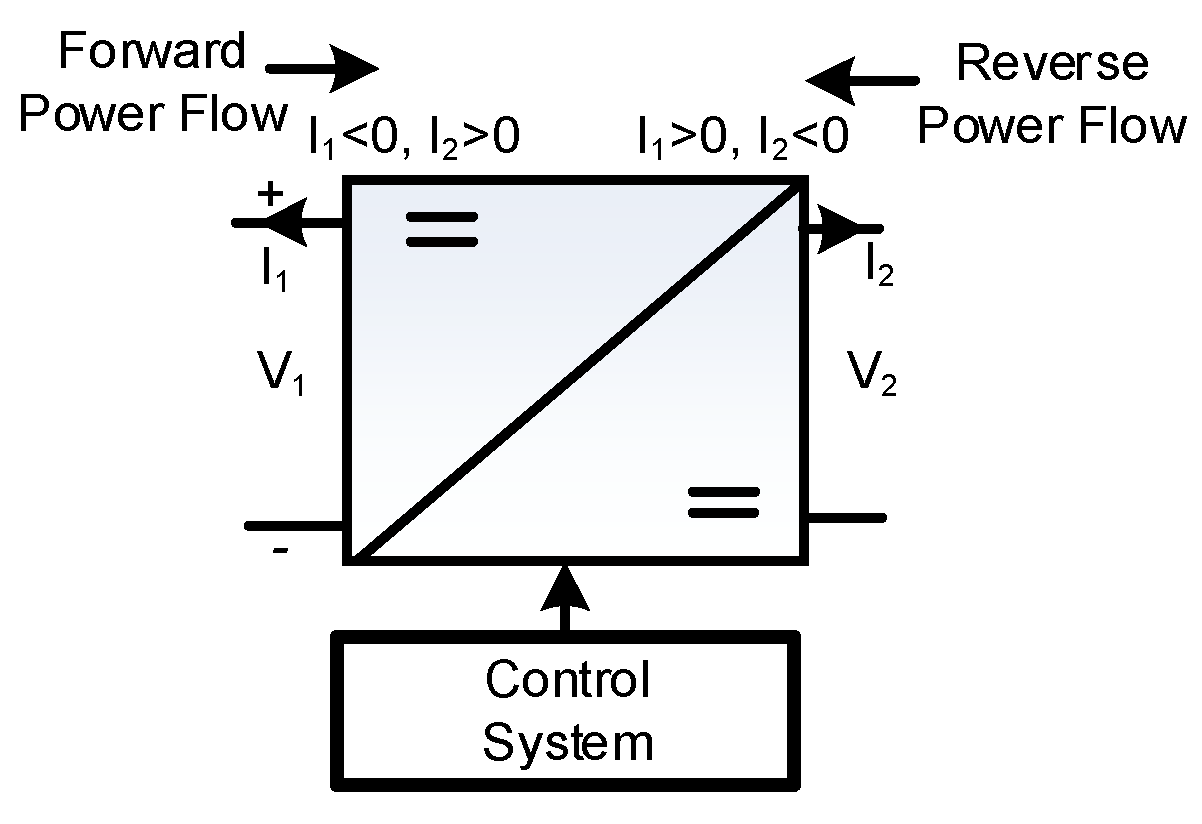Figure 2. Conventional bidirectional converter (BDC).
Figure 2. Conventional bidirectional converter (BDC).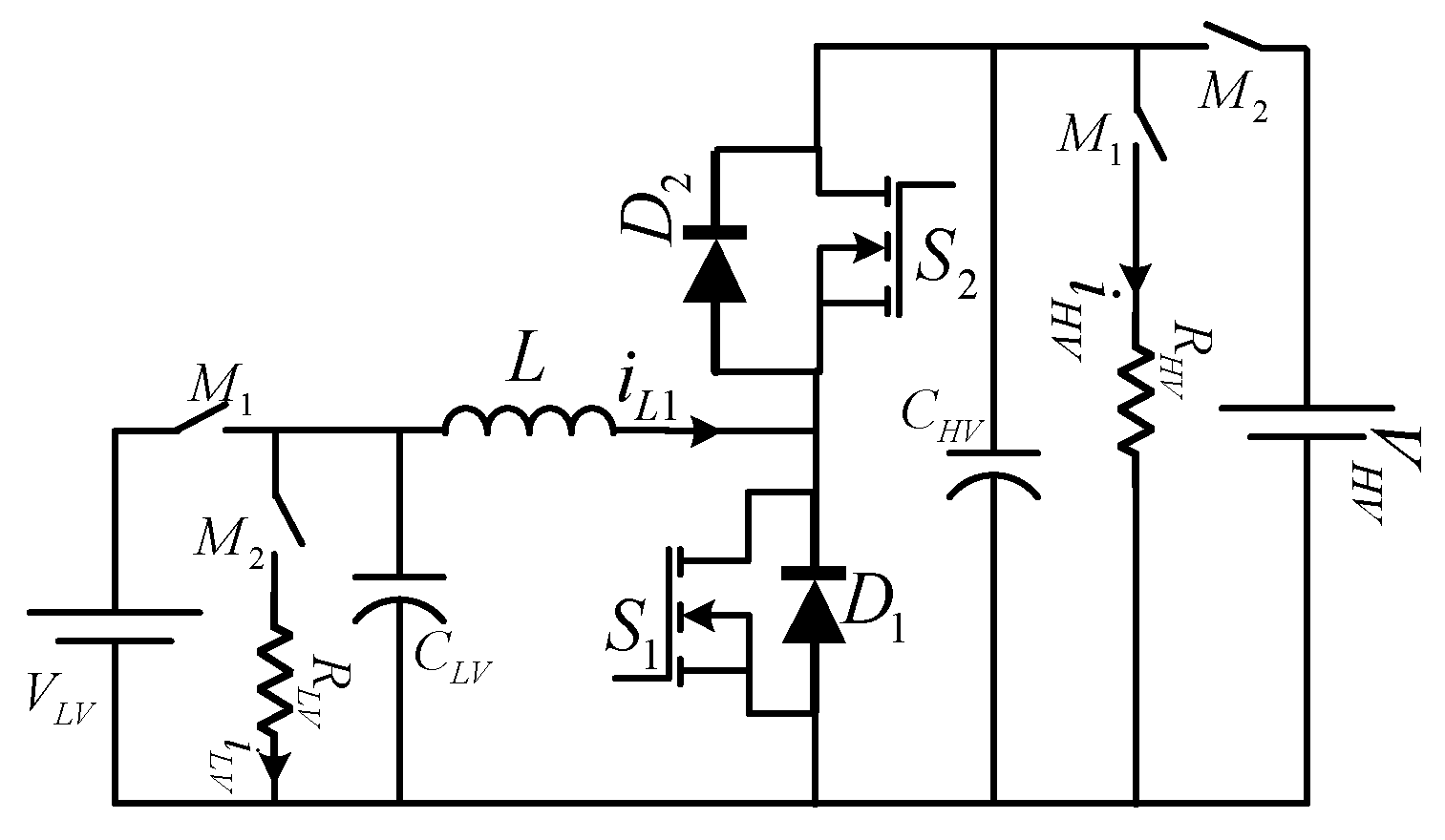Figure 3. Impedance DC–DC converters. (a) Z-source DC–DC converter, (b) switched boost DC–DC converter.
Figure 3. Impedance DC–DC converters. (a) Z-source DC–DC converter, (b) switched boost DC–DC converter.Figure 4. LC impedance bi-directional DC–DC converter (LC-BDC).
Figure 4. LC impedance bi-directional DC–DC converter (LC-BDC).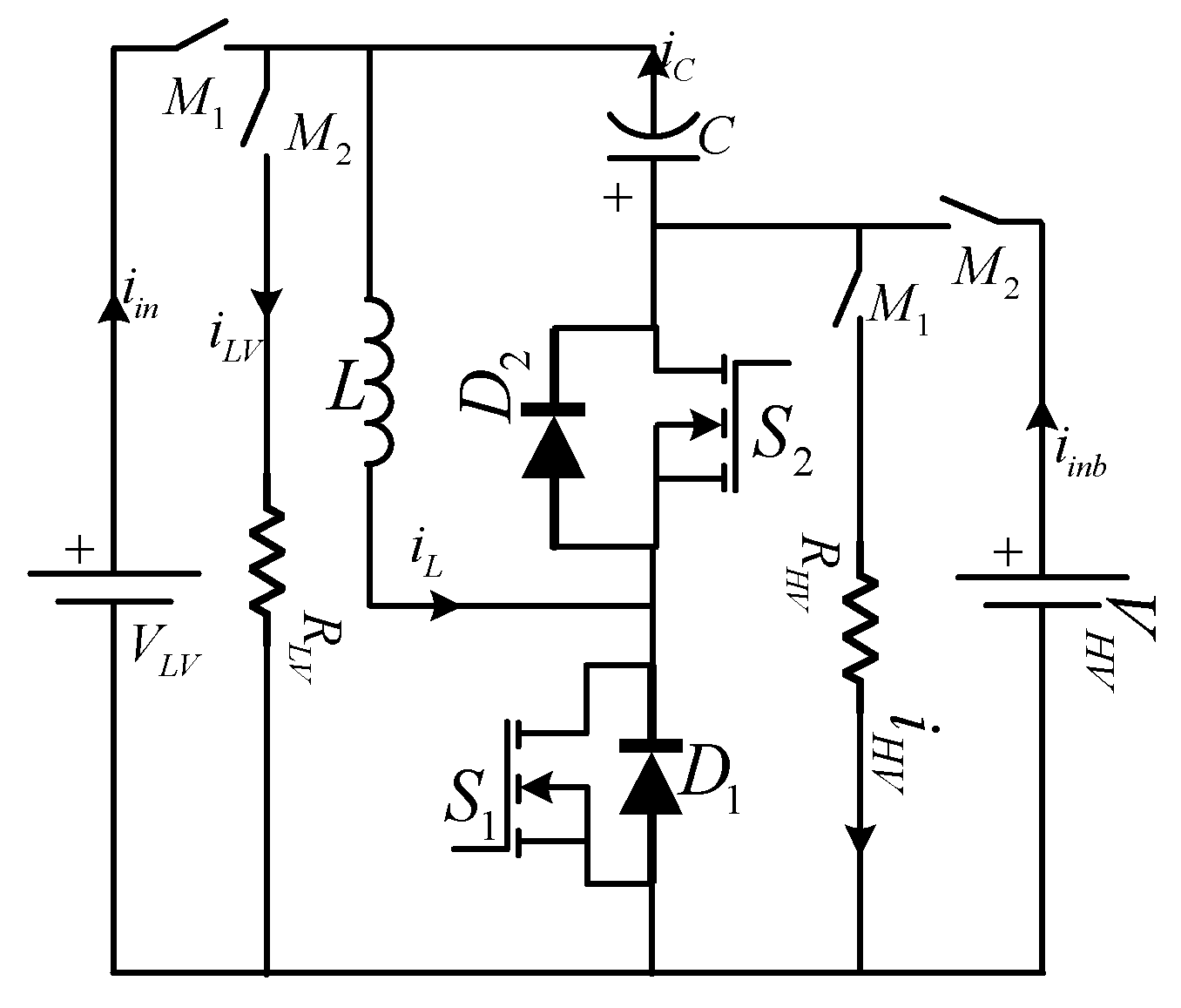Figure 5. LC bi-directional DC–DC converter in boost mode.
Figure 5. LC bi-directional DC–DC converter in boost mode.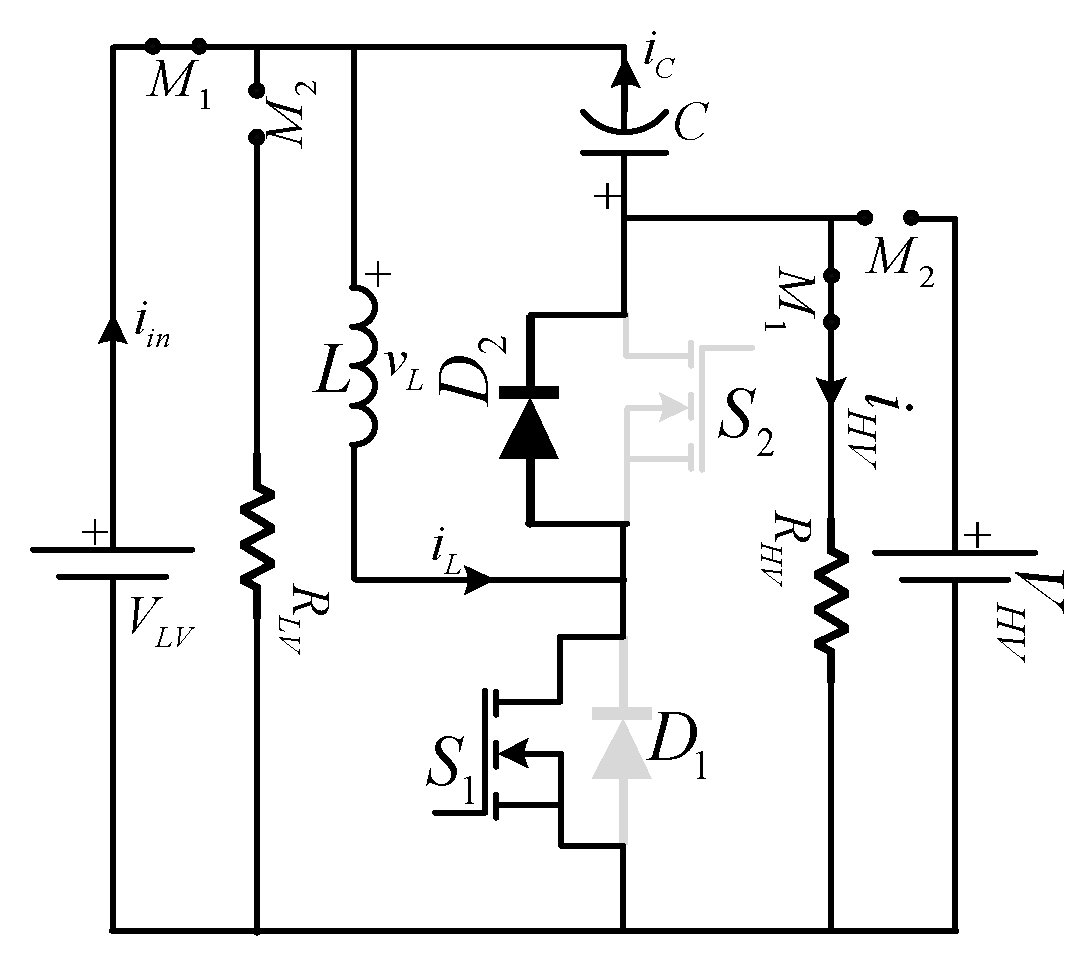Figure 6. Characteristic waveforms during various boost modes of operation.
Figure 6. Characteristic waveforms during various boost modes of operation.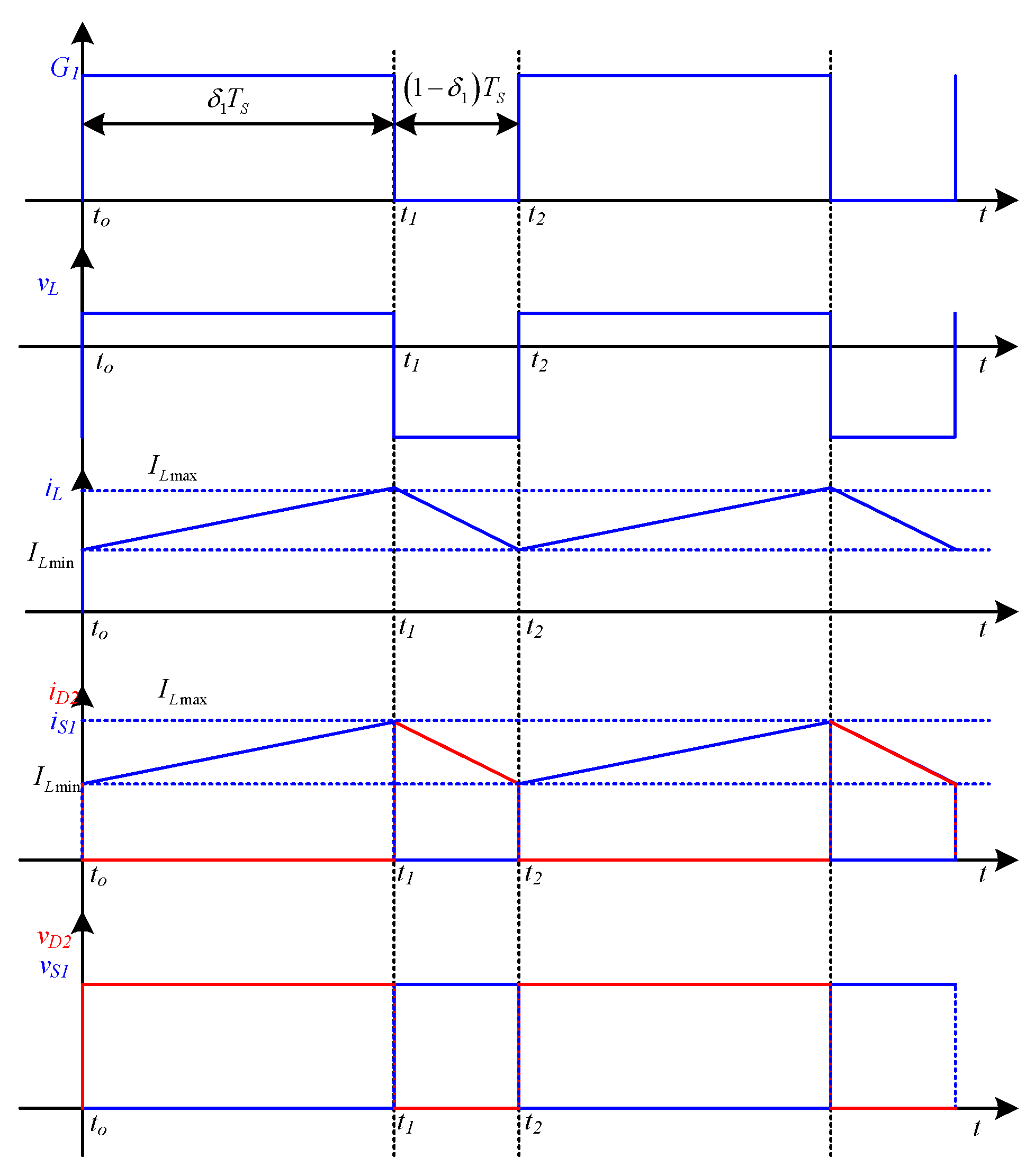Figure 7. Equivalent circuit of boost operation (a) in mode 1 and (b) in mode 2.
Figure 7. Equivalent circuit of boost operation (a) in mode 1 and (b) in mode 2.Figure 8. Equivalent circuit in buck mode.
Figure 8. Equivalent circuit in buck mode.Figure 9. Characteristic waveforms during various modes in buck operation.
Figure 9. Characteristic waveforms during various modes in buck operation.Figure 10. Equivalent circuit of buck operation (a) in mode 3 and (b) in mode 4.
Figure 10. Equivalent circuit of buck operation (a) in mode 3 and (b) in mode 4.Figure 11. Step responses of the inductor current, output voltage, load current, and capacitor voltage transfer functions with respect to duty ratio and input voltage of LC-BDC and conventional converter.
Figure 11. Step responses of the inductor current, output voltage, load current, and capacitor voltage transfer functions with respect to duty ratio and input voltage of LC-BDC and conventional converter.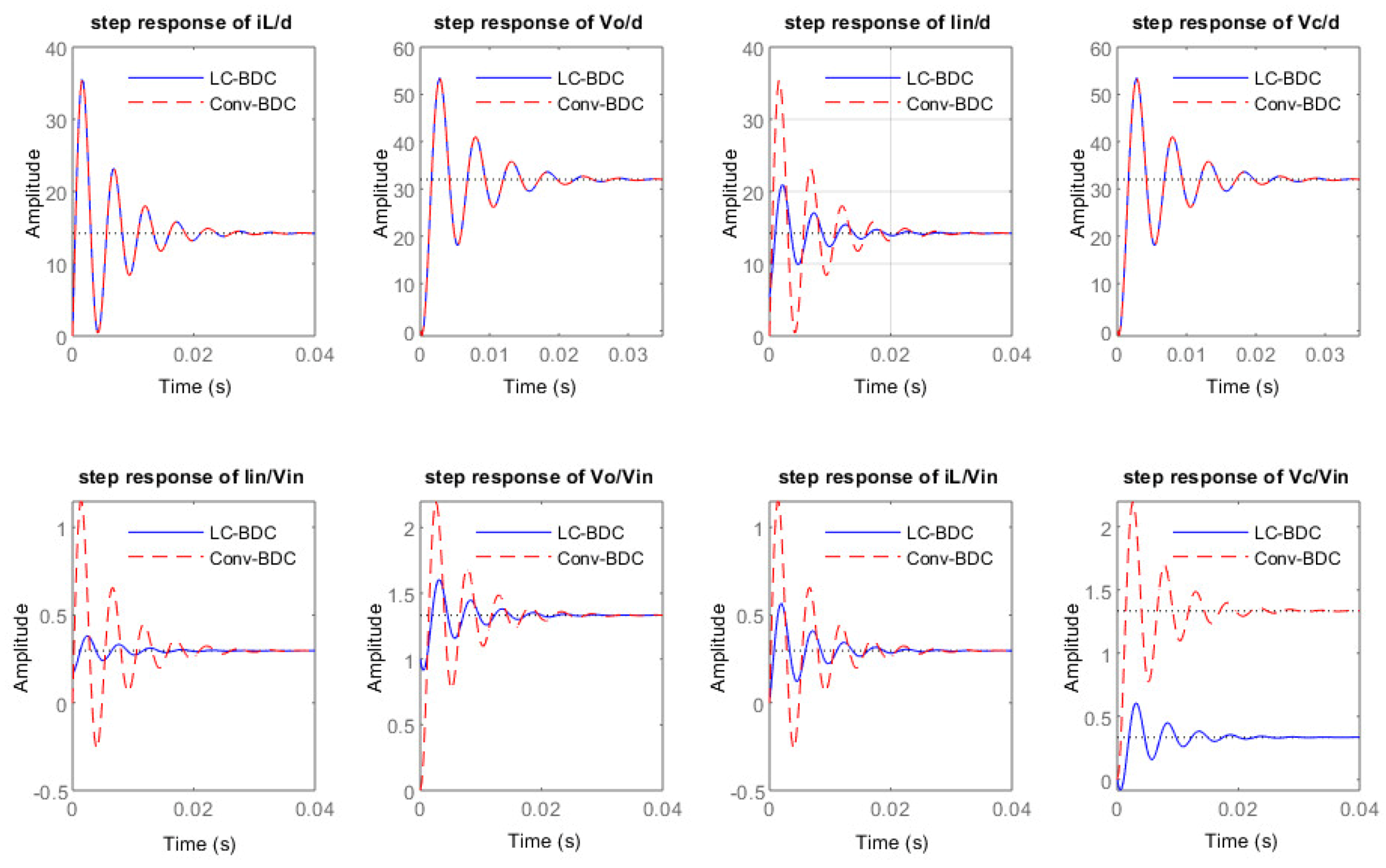Figure 12. Experimental Setup of Power Converter.
Figure 12. Experimental Setup of Power Converter.Figure 13. Gate voltage of lower switch (S1) in LC-BDC (Blue), and conventional BDC (red).
Figure 13. Gate voltage of lower switch (S1) in LC-BDC (Blue), and conventional BDC (red).Figure 14. Inductor current in LC-BDC (Blue), and conventional BDC (red).
Figure 14. Inductor current in LC-BDC (Blue), and conventional BDC (red).Figure 15. Load current in LC-BDC (Blue), and conventional BDC (red).
Figure 15. Load current in LC-BDC (Blue), and conventional BDC (red).Figure 16. Capacitor voltages in LC-BDC (Blue), and conventional BDC (Red).
Figure 16. Capacitor voltages in LC-BDC (Blue), and conventional BDC (Red).Figure 17. Inductor current of LC-BDC (blue) and conventional BDC (red) converter during (a) “on” and (b) “off” states.
Figure 17. Inductor current of LC-BDC (blue) and conventional BDC (red) converter during (a) “on” and (b) “off” states.Figure 18. Load current of LC-BDC (blue) and conventional BDC (red) converter during (a) “on” and (b) “off” states.
Figure 18. Load current of LC-BDC (blue) and conventional BDC (red) converter during (a) “on” and (b) “off” states.Figure 19. Capacitor voltage of LC-BDC (blue) and conventional BDC (red) converter during (a) “on” and (b) “off” states.
Figure 19. Capacitor voltage of LC-BDC (blue) and conventional BDC (red) converter during (a) “on” and (b) “off” states.Figure 20. Zoomed view of inductor current for ripple analysis LC-BDC (blue), and conventional converter (red).
Figure 20. Zoomed view of inductor current for ripple analysis LC-BDC (blue), and conventional converter (red).Figure 21. Zoomed view of load current for ripple analysis in LC-BDC (blue), and conventional converter (red).
Figure 21. Zoomed view of load current for ripple analysis in LC-BDC (blue), and conventional converter (red).Figure 22. Zoomed view of capacitor voltage in the conventional converter for ripple analysis.
Figure 22. Zoomed view of capacitor voltage in the conventional converter for ripple analysis.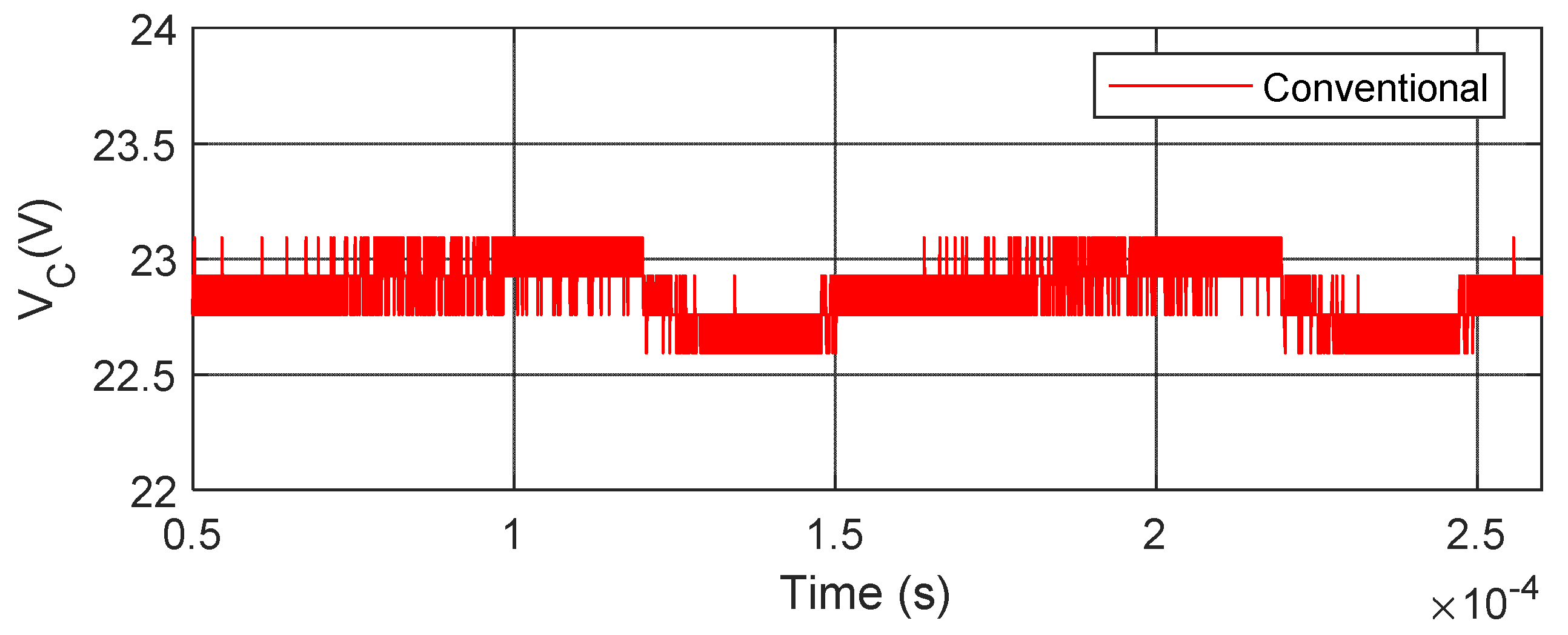Figure 23. Zoomed view of capacitor voltage in the proposed converter for ripple analysis.
Figure 23. Zoomed view of capacitor voltage in the proposed converter for ripple analysis.Figure 24. Gate voltage of upper switch (S2) in LC-BDC (Blue), and conventional BDC (red).
Figure 24. Gate voltage of upper switch (S2) in LC-BDC (Blue), and conventional BDC (red).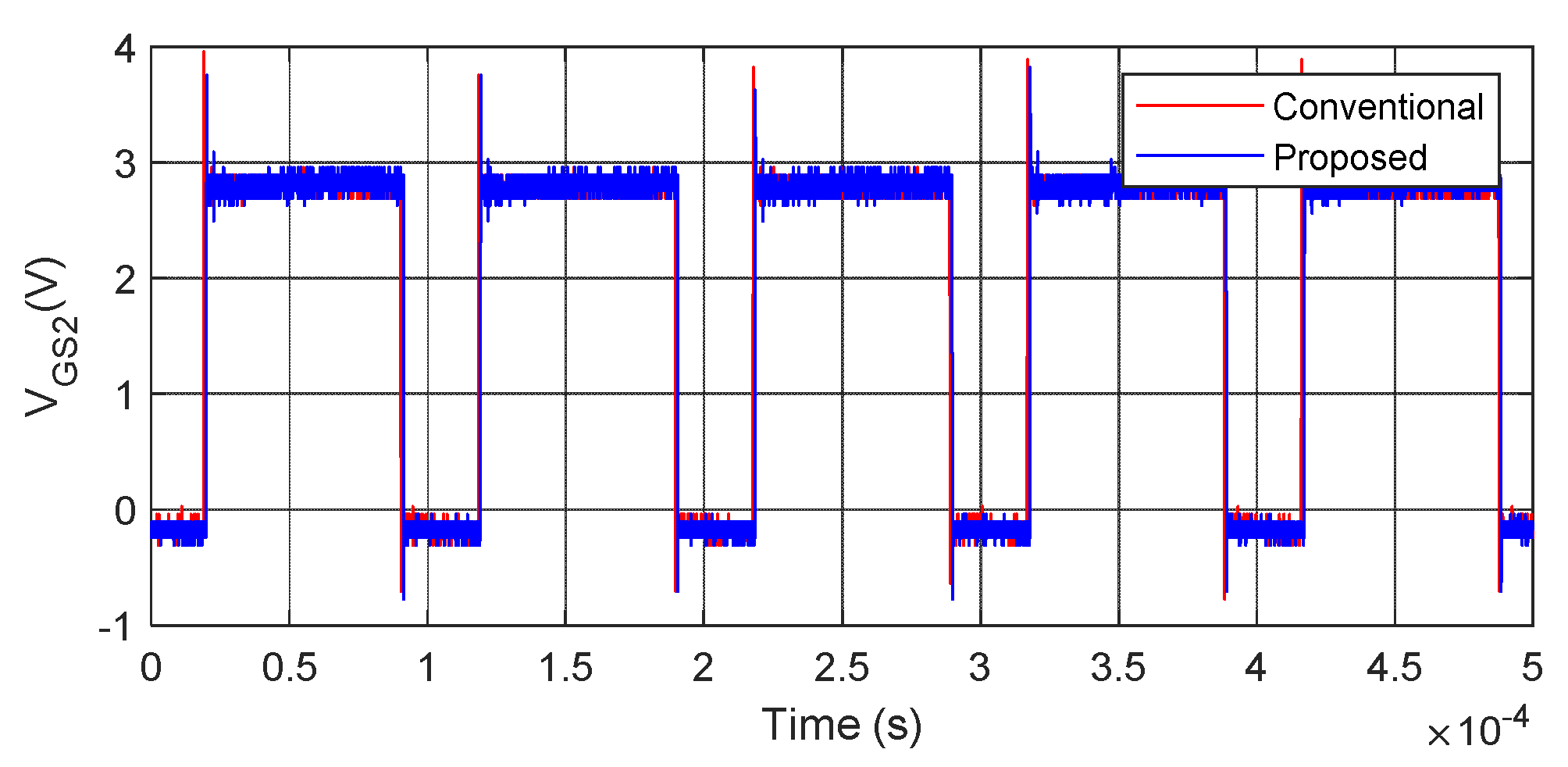Figure 25. Inductor current in LC-BDC (Blue), and conventional BDC (red).
Figure 25. Inductor current in LC-BDC (Blue), and conventional BDC (red).Figure 26. Load current in LC-BDC (Blue), and conventional BDC (red).
Figure 26. Load current in LC-BDC (Blue), and conventional BDC (red).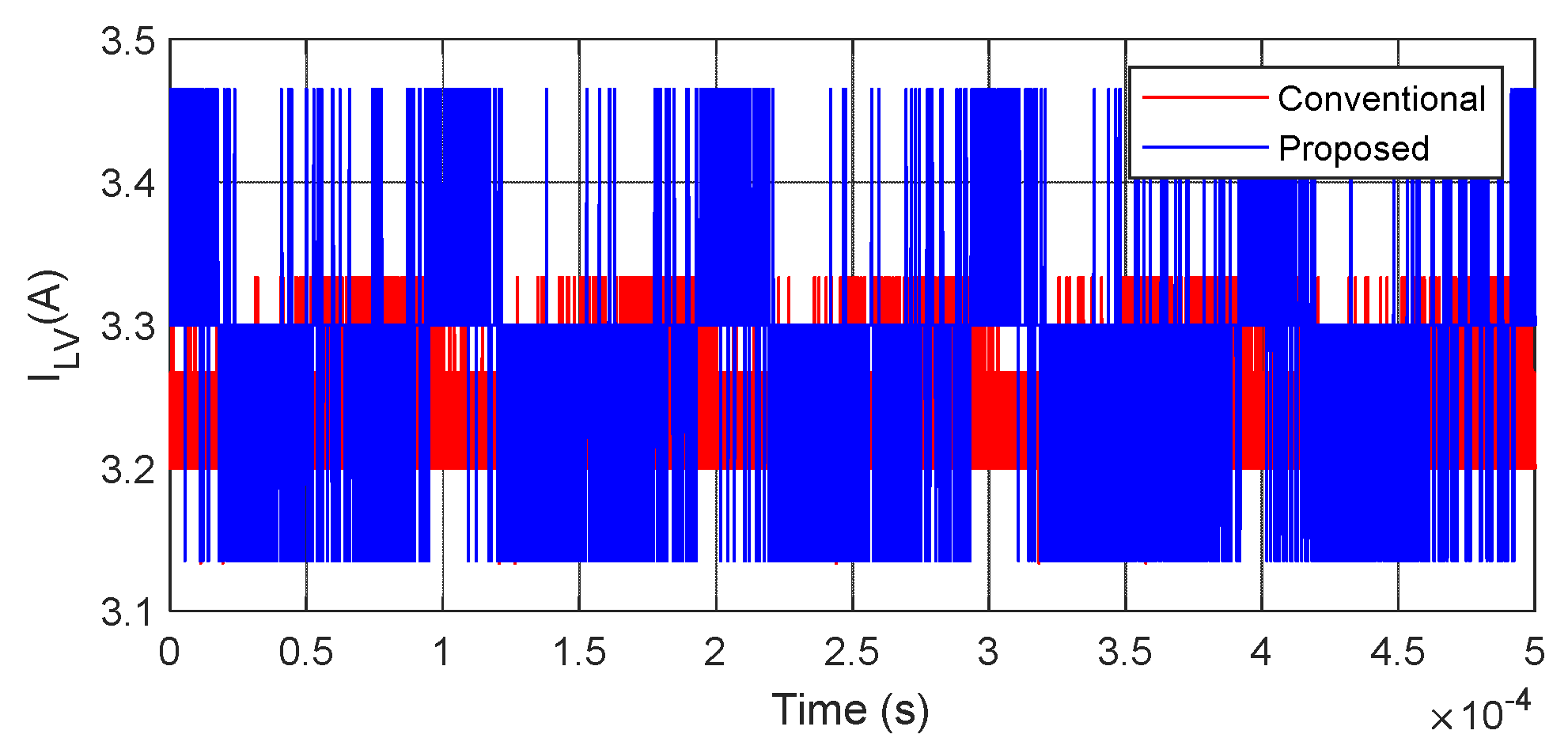Figure 27. Capacitor voltages in LC-BDC (Blue), and conventional BDC (red).
Figure 27. Capacitor voltages in LC-BDC (Blue), and conventional BDC (red).Figure 28. Inductor current of LC-BDC (blue) and conventional BDC (red) converter during (a) turn on (b) turn off.
Figure 28. Inductor current of LC-BDC (blue) and conventional BDC (red) converter during (a) turn on (b) turn off.Figure 29. Load current of LC-BDC (blue) and conventional BDC (red) converter during (a) turn on and (b) turn off.
Figure 29. Load current of LC-BDC (blue) and conventional BDC (red) converter during (a) turn on and (b) turn off.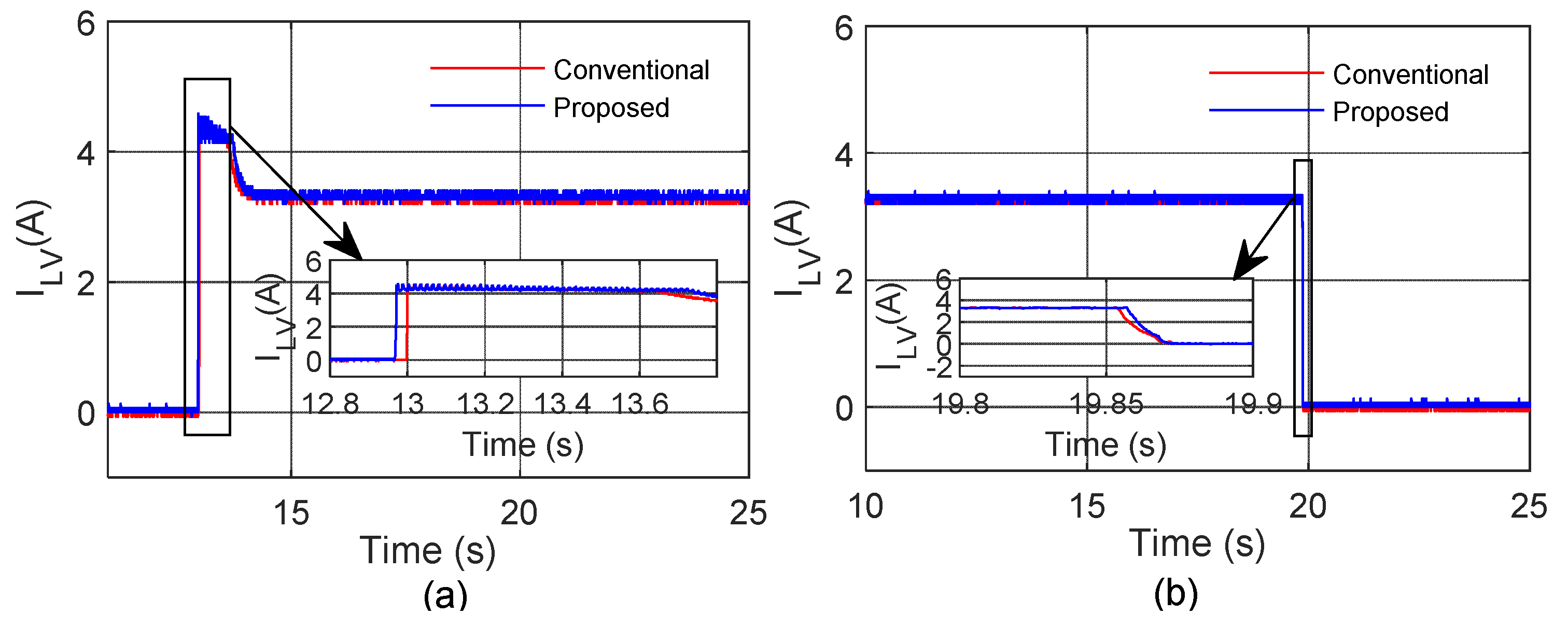Figure 30. Capacitor voltage of LC-BDC (blue) and conventional BDC (red) converter during (a) turn on and (b) turn off.
Figure 30. Capacitor voltage of LC-BDC (blue) and conventional BDC (red) converter during (a) turn on and (b) turn off.Figure 31. Zoomed view of inductor current for ripple analysis LC-BDC (blue), and conventional converter (red).
Figure 31. Zoomed view of inductor current for ripple analysis LC-BDC (blue), and conventional converter (red).Figure 32. Zoomed view of load current for ripple analysis in LC-BDC (blue), and conventional converter (red).
Figure 32. Zoomed view of load current for ripple analysis in LC-BDC (blue), and conventional converter (red).Figure 33. Zoomed view of capacitor voltage in the conventional converter for ripple analysis.
Figure 33. Zoomed view of capacitor voltage in the conventional converter for ripple analysis.Figure 34. Zoomed view of capacitor voltage in the proposed converter for ripple analysis.
Figure 34. Zoomed view of capacitor voltage in the proposed converter for ripple analysis.Figure 35. Bar chart of no. of components used in different topology.
Figure 35. Bar chart of no. of components used in different topology.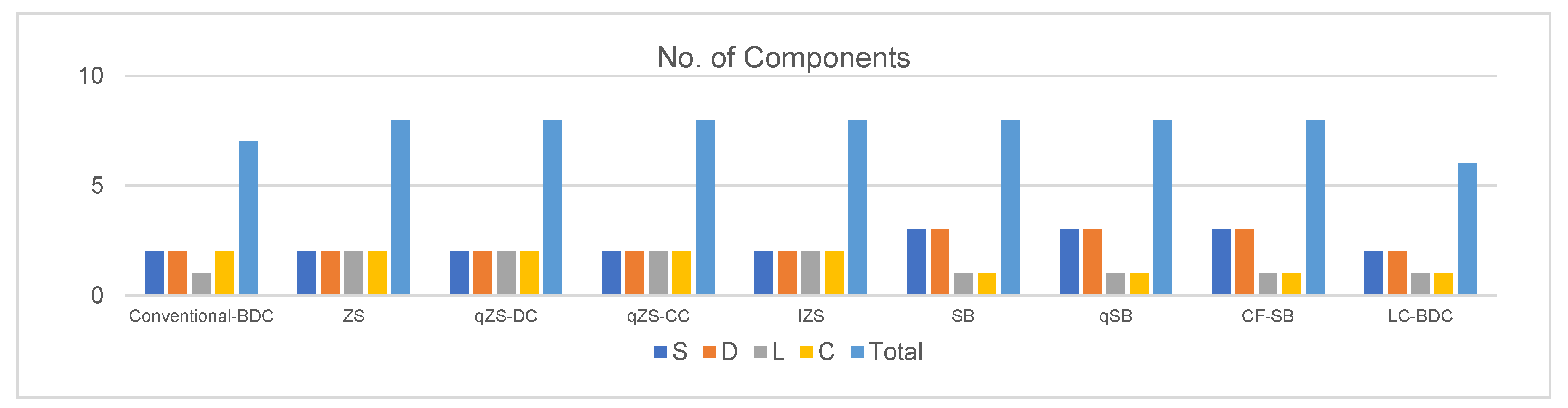Figure 36. Total capacitor stress in different topology.
Figure 36. Total capacitor stress in different topology.Figure 37. Efficiency comparison of various topologies.
Figure 37. Efficiency comparison of various topologies.Figure 38. Voltage conversion ratio comparison of various topologies.
Figure 38. Voltage conversion ratio comparison of various topologies.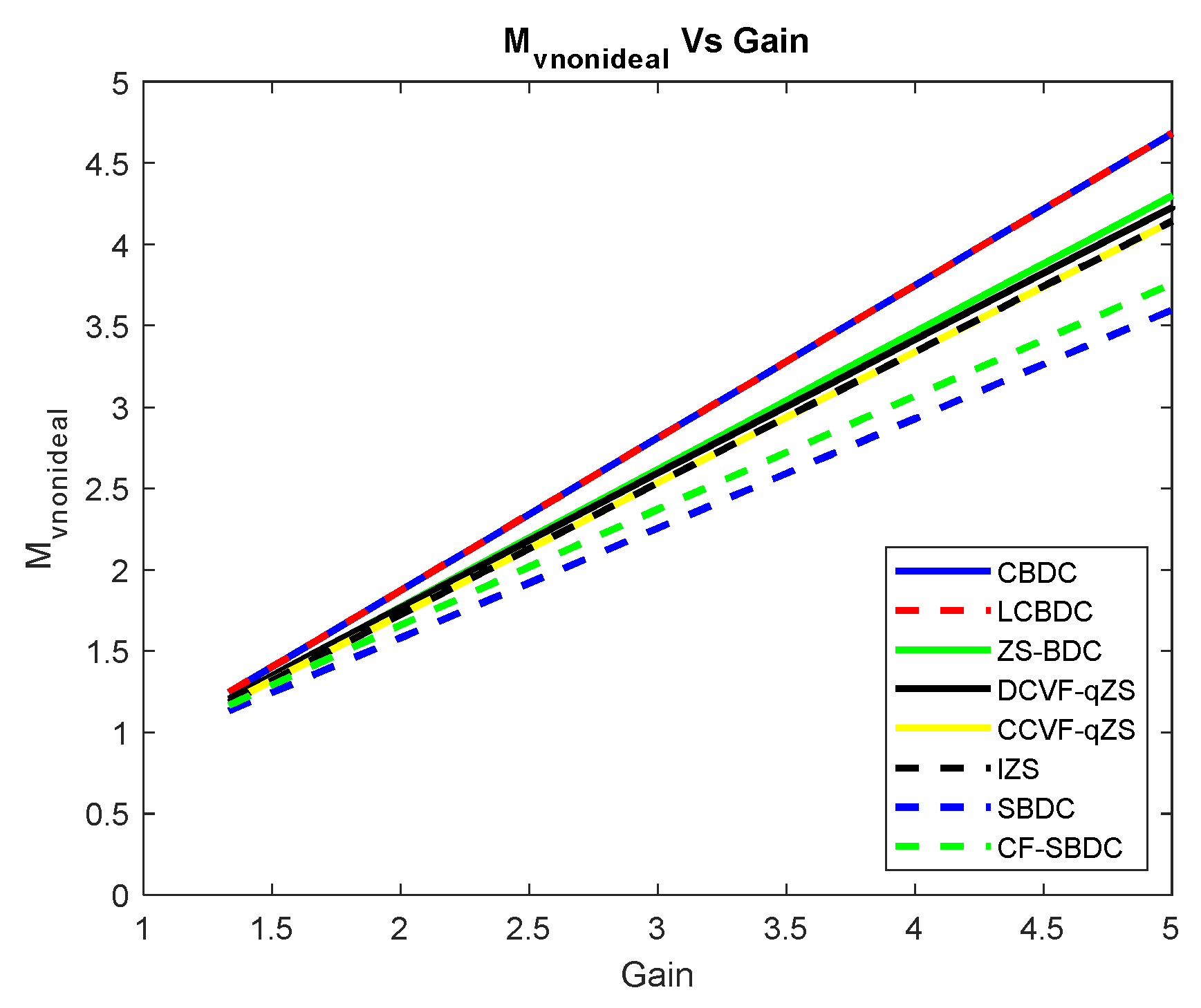Table 1. Parameters of the proposed converter.
Table 1. Parameters of the proposed converter.
Proposed Conventional
Parameter nameBoost Mode (LV to HV)Buck Mode (HV to LV)Boost Mode (LV to HV)Buck Mode (HV to LV)
Input voltage (V)18241824
Output voltage (V)24182418
Output voltage ripple, %$≤$0.50$≤$0.50$≤$0.50$≤$0.50
Load12 V, 50 W of 2 Lamps in series12 V, 50 W of 2 Lamps in series12 V, 50 W of 2 Lamps in series12 V, 50 W of 2 Lamps in series
Output current (A)43.2043.20
Switching frequency (kHz)10101010
Inductor
Filter capacitors(HV Side)
(LV Side)

(intermediate stage)
Table 2. Comparison of the various parameter during both transient and steady state for the proposed and conventional converters.
Table 2. Comparison of the various parameter during both transient and steady state for the proposed and conventional converters.
Peak ValuesConventionalProposed
Capacitor voltage23.20 V23.20 V4.80 V5.20 V
Inductor current8.80 A6.00 A8.80 A6.00 A
Load Current5.20 A4.00 A5.20 A4.00 A
Table 3. Comparison of ripple values of capacitor voltage, inductor current and load current for the proposed and conventional converters.
Table 3. Comparison of ripple values of capacitor voltage, inductor current and load current for the proposed and conventional converters.
RipplesConventionalProposed
Capacitor voltage0.50 V0.34 V
Input current0.99 A0.79 A
Table 4. Comparison of the various parameter during both transient and steady states for the proposed and conventional converters.
Table 4. Comparison of the various parameter during both transient and steady states for the proposed and conventional converters.
Peak ValuesConventionalProposed
Capacitor voltage5.60 V16.40 V6.20 V7.80 V
Inductor current4.54 A3.65 A4.62 A3.68 A
Load Current4.54 A3.65 A4.62 A3.68 A
Table 5. Comparison of Ripple Values for The Proposed and Conventional Converters in Buck Mode.
Table 5. Comparison of Ripple Values for The Proposed and Conventional Converters in Buck Mode.
RipplesConventionalProposed
Capacitor voltage1.40%1.10%
Inductor current16.65%16.65%
Table 6. Parameters considered for comparative analysis.
Table 6. Parameters considered for comparative analysis.
ParameterValue
Input DC Voltage 24 V
Switching Frequency10 kHz
Output line voltage (RMS)36 V–108 V
Table 7. Comparison of various parameters (device, inductor and capacitor RMS currents, overall losses, and efficiency) of existing and proposed topologies.
Table 7. Comparison of various parameters (device, inductor and capacitor RMS currents, overall losses, and efficiency) of existing and proposed topologies.
TopologyEquivalent Circuit DiagramRMS Currents of Various ComponentsParameters
Conv-BDC$I s r m s = I o D 1 − D$
$I D r m s = I o 1 − D$
$I L r m s = I o 1 − D$
$I C r m s = I o D 1 − D$
$η = 1 1 + { r L + D r D S ( 1 − D ) 2 R L + R F + D r c ( 1 − D ) R L + V f V o + F s C o R L }$
$M V D S = 1 ( 1 − D ) { r L + D r D S ( 1 − D ) 2 R L + R F + D r c ( 1 − D ) R L + V f V o + F s C o R L }$
LC-BDC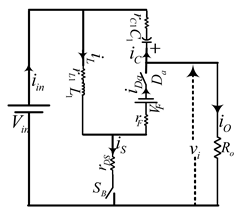$I S r m s = I o D 1 − D$
$I D r m s = I o 1 − D$
$I L r m s = I o 1 − D$
$I C r m s = I o D 1 − D$
$η = 1 1 + { r L + D r D S ( 1 − D ) 2 R L + R F + D r c ( 1 − D ) R L + V f V o + F s C o R L }$
$M V D S = 1 ( 1 − D ) { r L + D r D S ( 1 − D ) 2 R L + R F + D r c ( 1 − D ) R L + V f V o + F s C o R L }$
ZS-BDC$I S r m s = I o D 1 − 2 D$
$I D r m s = ( 1 − D ) 3 / 2 ( 1 − 2 D ) I o$
$I L r m s = 1 − D 1 − 2 D I o$
$I C r m s = D ( 1 − D ) ( 1 − 2 D ) I o$
$η = 1 1 + { D r D s ( 1 − 2 D ) 2 R L + ( 1 − D ) 2 ( 1 − 2 D ) V f V o + ( 1 − D ) 3 ( 1 − 2 D ) 2 R F R L + + 2 ( 1 − D 1 − 2 D ) 2 r L R L + F s C o R L 2 + r L F R L + 2 D ( 1 − D ) ( 1 − 2 D ) 2 r c R L + ( M V d c − 1 ) 2 12 ( L f F s ) 2 ( 1 − 2 D ) 2 r C F R L }$
$M V D S = ( 1 − D ) ( 1 − 2 D ) { D r D s ( 1 − 2 D ) 2 R L + ( 1 − D ) 2 ( 1 − 2 D ) V f V o + ( 1 − D ) 3 ( 1 − 2 D ) 2 R F R L + + 2 ( 1 − D 1 − 2 D ) 2 r L R L + F s C o R L 2 + r L F R L + 2 D ( 1 − D ) ( 1 − 2 D ) 2 r c R L + ( M V d c − 1 ) 2 12 ( L f F s ) 2 ( 1 − 2 D ) 2 r C F R L }$
DCVF-qZS BDC$I S r m s = I o D 1 − 2 D$
$I D r m s = 1 − D I o$
$I L r m s = 1 − D 1 − 2 D I o$
$I C r m s = D ( 1 − D ) ( 1 − 2 D ) I o$
$η = 1 1 + { D r D s ( 1 − 2 D ) 2 R L + ( 1 − D ) V f V o + ( 1 − D ) 2 R F ( 1 − D ) R L + 2 ( 1 − D 1 − 2 D ) 2 r L R L + F s C o R L 2 + r L F R L + ( M V d c − 1 ) 2 12 ( L f F s ) 2 ( 1 − 2 D ) 2 r C F R L + 2 D ( 1 − D ) ( 1 − 2 D ) 2 r c R L }$
$M V D S = ( 1 − D ) ( 1 − 2 D ) { D r D s ( 1 − 2 D ) 2 R L + ( 1 − D ) V f V o + ( 1 − D ) 2 R F ( 1 − D ) R L + 2 ( 1 − D 1 − 2 D ) 2 r L R L + F s C o R L 2 + r L F R L + ( M V d c − 1 ) 2 12 ( L f F s ) 2 ( 1 − 2 D ) 2 r C F R L + 2 D ( 1 − D ) ( 1 − 2 D ) 2 r c R L }$
CCVF-qZS BDC$I S r m s = I o D 1 − 2 D$
$I D r m s = 1 − D ( 1 − 2 D ) I o$
$I L r m s = 1 − D 1 − 2 D I o$
$I C r m s = D ( 1 − D ) ( 1 − 2 D ) I o$
$η = 1 1 + { D r D s ( 1 − 2 D ) 2 R L + ( 1 − D ) ( 1 − 2 D ) V f V o + ( 1 − D ) ( 1 − 2 D ) 2 R F R L + F s C o R L 2 + r L F R L + 2 ( 1 − D 1 − 2 D ) 2 r L R L + 2 D ( 1 − D ) ( 1 − 2 D ) 2 r c R L + ( M V d c − 1 ) 2 12 ( L f F s ) 2 ( 1 − 2 D ) 2 r C F R L }$
$M V D S = ( 1 − D ) ( 1 − 2 D ) { D r D s ( 1 − 2 D ) 2 R L + ( 1 − D ) ( 1 − 2 D ) V f V o + ( 1 − D ) ( 1 − 2 D ) 2 R F R L + F s C o R L 2 + r L F R L + 2 ( 1 − D 1 − 2 D ) 2 r L R L + 2 D ( 1 − D ) ( 1 − 2 D ) 2 r c R L + ( M V d c − 1 ) 2 12 ( L f F s ) 2 ( 1 − 2 D ) 2 r C F R L }$
IZS-BDC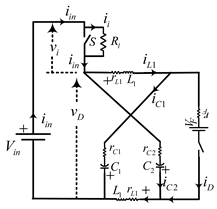$I S r m s = I o D 1 − 2 D$
$I D r m s = ( 1 − D ) 1 / 2 ( 1 − 2 D ) I o$
$I L r m s = 1 − D 1 − 2 D I o$
$I C r m s = D ( 1 − D ) ( 1 − 2 D ) I o$
$η = 1 1 + { D r D s ( 1 − 2 D ) 2 R L + ( 1 − D ) ( 1 − 2 D ) V f V o + ( 1 − D ) ( 1 − 2 D ) 2 R F R L + F s C o R L 2 + r L F R L + 2 ( 1 − D 1 − 2 D ) 2 r L R L + 2 D ( 1 − D ) ( 1 − 2 D ) 2 r c R L + ( M V d c − 1 ) 2 12 ( L f F s ) 2 ( 1 − 2 D ) 2 r C F R L } P o$
$M V D S = ( 1 − D ) ( 1 − 2 D ) { D r D s ( 1 − 2 D ) 2 R L + ( 1 − D ) ( 1 − 2 D ) V f V o + ( 1 − D ) ( 1 − 2 D ) 2 R F R L + F s C o R L 2 + r L F R L + 2 ( 1 − D 1 − 2 D ) 2 r L R L + 2 D ( 1 − D ) ( 1 − 2 D ) 2 r c R L + ( M V d c − 1 ) 2 12 ( L f F s ) 2 ( 1 − 2 D ) 2 r C F R L } P o$
SB-BDC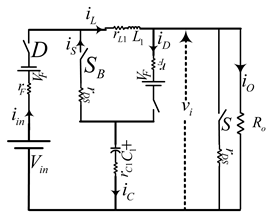$I S r m s = I o D 1 − 2 D$
$I D r m s = ( 1 − D ) 1 / 2 ( 1 − 2 D ) I o$
$I L r m s = 1 1 − 2 D I o$
$I C r m s = 2 D ( 1 − D ) ( 1 − 2 D ) I o$
$η = 1 1 + { 2 D r D s ( 1 − 2 D ) 2 R L + ( 1 − D ) ( 1 − 2 D ) 2 V f V o + ( 1 − D ) ( 1 − 2 D ) 2 2 R F R L + F s C o R L + ( 1 1 − 2 D ) 2 r L R L + 4 D ( 1 − D ) ( 1 − 2 D ) 2 r c R L } P o$
$M V D S = ( 1 − D ) ( 1 − 2 D ) { 2 D r D s ( 1 − 2 D ) 2 R L + ( 1 − D ) ( 1 − 2 D ) 2 V f V o + ( 1 − D ) ( 1 − 2 D ) 2 2 R F R L + F s C o R L + ( 1 1 − 2 D ) 2 r L R L + 4 D ( 1 − D ) ( 1 − 2 D ) 2 r c R L } P o$
CFSB-BDC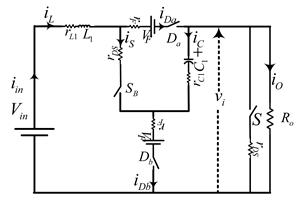$I S r m s = I o D 1 − 2 D$
$I D r m s = ( 1 − D ) 1 / 2 ( 1 − 2 D ) I o$
$I L r m s = 1 1 − 2 D I o$
$I C r m s = 2 D ( 1 − D ) ( 1 − 2 D ) I o$
$η = 1 1 + { 2 D r D s ( 1 − 2 D ) 2 R L + ( 1 − D ) ( 1 − 2 D ) 2 V f V o + ( 1 − D ) ( 1 − 2 D ) 2 2 R F R L + F s C o R L + ( 1 1 − 2 D ) 2 r L R L + 4 D ( 1 − D ) ( 1 − 2 D ) 2 r c R L } P o$
$M V D S = ( 1 − D ) ( 1 − 2 D ) { 2 D r D s ( 1 − 2 D ) 2 R L + ( 1 − D ) ( 1 − 2 D ) 2 V f V o + ( 1 − D ) ( 1 − 2 D ) 2 2 R F R L + F s C o R L + ( 1 1 − 2 D ) 2 r L R L + 4 D ( 1 − D ) ( 1 − 2 D ) 2 r c R L } P o$